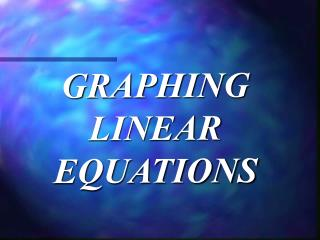DownloadDownload PresentationGRAPHING LINEAR EQUATIONS

# GRAPHING LINEAR EQUATIONS

Télécharger la présentation## GRAPHING LINEAR EQUATIONS

- - - - - - - - - - - - - - - - - - - - - - - - - - - E N D - - - - - - - - - - - - - - - - - - - - - - - - - - -
##### Presentation Transcript

1. GRAPHING LINEAR EQUATIONS

2. y2 - y1 m = x2 - x1 SLOPE FORMULA Given two points: (x1,y1) and (x2,y2)

3. FORMS OF LINEAR EQUATIONS

4. SLOPE INTERCEPT FORM y = m x + b m = slope b = y-intercept

5. A, B, and C are integers. A > 0 m = - A B STANDARD FORM Ax + By = C

6. POINT - SLOPE FORM y - y1 = m ( x - x1 ) m = slope the given point is (x1,y1)

7. GRAPHING 1. Choose wisely! 2. We need two things…. 3. We still need two things…. 4. We really need two things….

8. Graphing using Slope and y-intercept y = mx + b y = 2 x + 4 m = 2 y-intercept = 4

9. Graphing using Intercepts x y 0 0 Ax +By = C 3x + 4y = 12

10. y = 2x + 1 y 5 y 2x + 1 x 1 2 3 4 2(1) +1 2(2) +1 2(3) +1 2(4)+1 3 5 7 9 x 5 -5 - 5

11. Graphing using Intercepts (You must be joking!) x y 0 ? 0 Ax +By = C 3x + 7y = 12